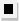Chapter 13.6, Problem 45E### Algebra and Trigonometry (MindTap ...

4th Edition
James Stewart + 2 others
ISBN: 9781305071742

#### Solutions

Chapter
Section### Algebra and Trigonometry (MindTap ...

4th Edition
James Stewart + 2 others
ISBN: 9781305071742
Textbook Problem

# 4 3 - 4 6Factoring Factor using the Binomial Theorem. 8 a 3 + 12 a 2 b + 6 a b 2 + b 3

To determine

To factor:

8a3+12a2b+6ab2+b3 using Binomial theorem.

Explanation

Given:

The expression 8a3+12a2b+6ab2+b3

Approach:

Deduce the exponent n of the binomial from number of terms in the given expression. And then compare the given expression to (x+y)n and solve for x,y.

Calculation:

The given expression has 4 terms and we know a binomial with exponent n has n+1 terms in its binomial expansion. Hence the binomial exponent corresponding to given expression is n=3

### Still sussing out bartleby?

Check out a sample textbook solution.

See a sample solution

#### The Solution to Your Study Problems

Bartleby provides explanations to thousands of textbook problems written by our experts, many with advanced degrees!

Get Started